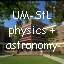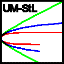# Wuzzlers in 1D Acceleration

Map-based relativity (i.e. relativity from the vantage point of a single space-time slice) allows one to solve constant acceleration problems at any speed, almost as easily as one might solve the Galilean constant acceleration problems common in today's introductory physics classes. It also makes for a nice transition from Newtonian physics, since Galileo's equations evolve naturally into the relativistic regime by simply asking the metric equation how moving clocks behave in context of a single reference map-frame. This list of mini-w{ebp}uzzlers, with equations, hints, and solutions where available, is meant to cover the full range of uni-directional constant proper-acceleration problem types. For some of these problems, closed-form solutions remain to be found. Maybe YOU can help!

no times given (avv, xvv, xav), one clock input (vv, av, xa, xv), two times known (a,v,x), given times only, solving tools, links

The (1+1)D motion integrals, in what is likely their most compact form, look like...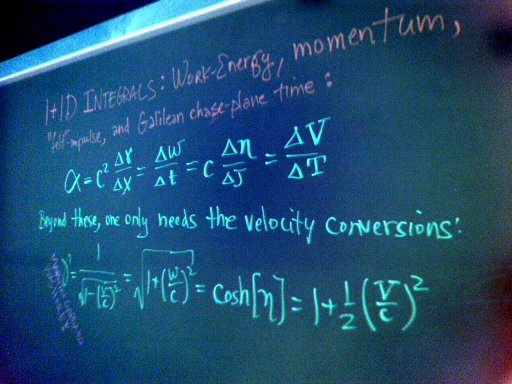For notation here, we use the "one-map, three-clocks" convention:
map-distance x, map-time t, coordinate-velocity v=dx/dt,
traveler-time tau, proper-velocity w=dx/dtau, hyperbolic velocity angle or rapidity eta,
plus Galilean-kinematic: chase-plane time T and velocity V=dx/dT.
Using c for lightspeed, velocity magnitudes obey γ > w/c > V/c > η > v/c,
while map-defined simultaneity yields time-increments such that Δt > ΔT > Δτ.

Here is a comprehensive look at the familiar Newtonian equations for unidirectional constant acceleration at low (i.e. non-relativistic) speed: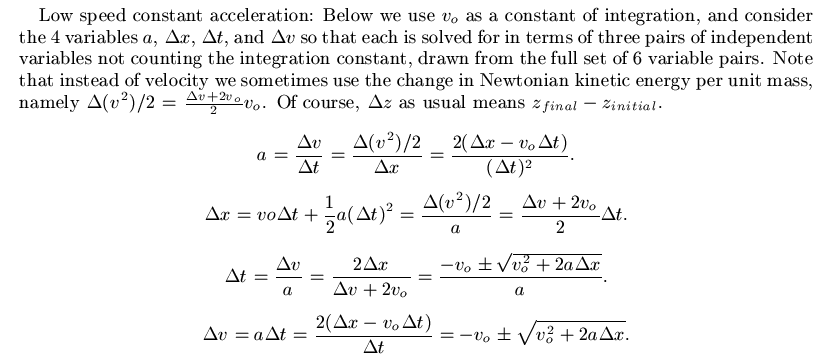The flat space metric equation, (cdτ)2 = (cdt)2 - (dx)2, requires that at high speeds we keep track of proper time τ as well as map-position x and time t. In addition to good old coordinate velocity v = dx/dt, we therefore must also consider proper velocity w = dx/dτ and "speed of map-time" γ = dt/dτ.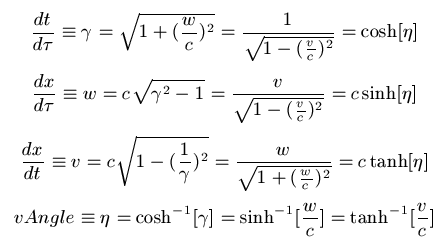That velocity angle (or rapidity) η in particular comes in handy when considering the motion of accelerated objects, following the strategy used for the low-speed equations above. In this case however, the acceleration of interest is the proper acceleration α experienced by the traveler, rather than the coordinate acceleration a = dv/dt.Multiplying parameters of the dashed curve below by mass
yields a family of kinetic energy versus momentum dispersion curves
that makes contact with much of everyday and modern physics.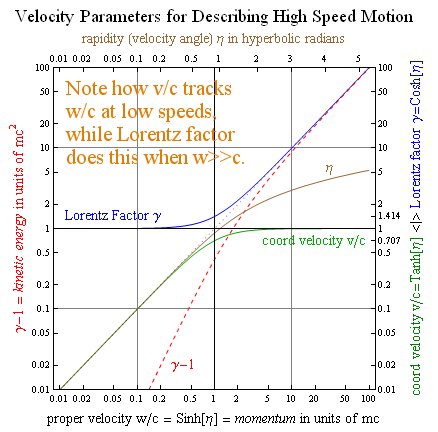A more comprehensive list of velocity inter-conversions is given below.At velocities small compared to lightspeed, parameters map most simply to the Galilean-kinematic,
with gamma becoming a version of E/mc^2 that uses Newton's kinetic energy expression,
and all other velocity parameters become either V or V/c.
In other words, gamma = 1 + (1/2)(V/c)2 and V ~ v ~ w ~ c eta.
The whole velocity-parameter conversion table thus simplifies at low speeds to...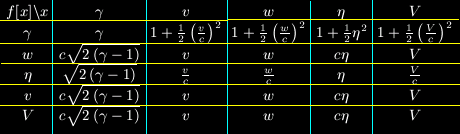As illustrated in this summary slide, the integrals of constant proper-acceleration
at low speeds also reduce to their Newtonian form: α ~ (1/2) Δ(v2)/Δx ~ Δv/Δt.

Here are a couple of recent plots about accelerated-twin round trips, seen from the perspective of a "stationary" spacetime slice. The first is a plot of velocity parameters and times on the horizontal axis, versus position on the vertical axis, for an accelerated roundtrip to a destination Δx a distance of 10c2/α away. If proper acceleration α~9.8 m/s2~1 ly/y2, then it corresponds to a destination 10 lightyears away. As you can see the traveler might be only 10 years older on return, even though almost 25 years have elapsed on earth.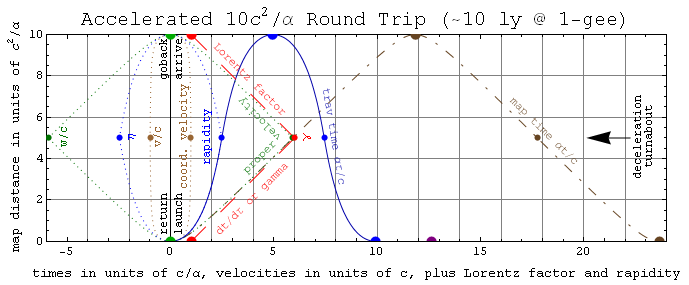This second plot shows how a spaceship able to accelerate/decelerate at 1 gee for 100 years could take you on a round trip to most anywhere in the visible universe, and bring you back home to earth in a lifetime or less. Additional time may have elapsed on earth by the time that you return, and of course building a spaceship that can do that is easier said than done. Although the small payload/launchmass ratios for rocket-propelled roundtrips are daunting, they suggest that a cleverly-designed spaceship with the mass of the space shuttle might deliver as many as a billion nannites (0.2 micron seeds) to dust clouds in the Andromeda galaxy, in map times much less than that required for intragalactic mixing. Much lower accelerations (even off-the-shelf ion-thrusters) might also work for nannite transport, since traveler time is less of an issue.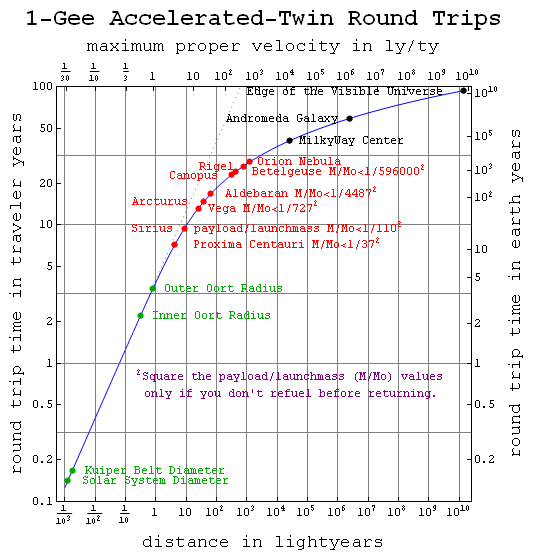New:
• 4/29/05 - A "live remote" platform for empirical study of the spacetime connection.
• Notes on anyspeed modeling, modernizing Newton, and one-map two-clocks
• More on the variables used here: this PDF or this summary slide.
• More broadly speaking, here's a roadmap to some local content modernization work.
• 10/01/97 - A Java (JDK1.1) applet version of our anyspeed solver.
• 9/24/97 - One way to solve for everything in an anyspeed acceleration problem.
• 9/16/97 - Three abstracts for the Jan 1998 winter AAPT meeting.
• 9/3/97 - A "vaccine on paper", to empower and protect introductory physics students!
• 4/30/97 - Excerpts from an any-speed motion primer with 1D-acceleration nomogram.• 3/01/97 - Start relativity with the metric equation instead of Lorentz transforms!.
• 2/28/97 - Invariance-colored 2-clock eqn tables: basic, 1d from rest, accel-3d, multi-map.
• 2/22/97 - Abstract on two-clock relativity for the April APS/AAPT conference in DC.
• 2/11/97 - Light-memes on 2-clock, 3-clock, metric-based, & magnet relativity.
• 1/22/97 - MathCAD worksheets (example snapshot) on 1D and 3D map-based relativity.
• 12/22/96 - A paper on "classy" ways to use Newton's laws at hi-speed.
• 7/23/96 - (3+1)D equations for constant acceleration in the simplest possible form.

## Problems with no elapsed times given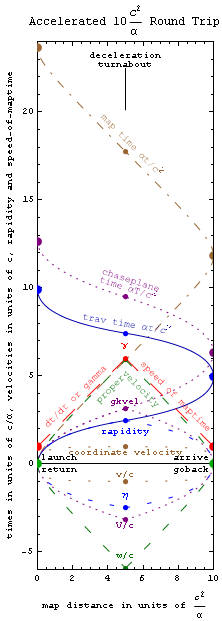### Given acceleration & intial/final velocities

• A rock thrown upward at 10 m/sec will go how far up? Hint. Ans. Soln[G/S].
• Map-time needed for a 1gee startup-burn to 0.9 c? Hint. Ans. Soln[G/S].
• Traveler-time needed for a 1gee retro-stop from 2 rb? Hint. Ans. Soln[G/S].

### Given distance and initial/final velocities?

• Acceleration for a 0-120 mph quarter mile? Hint. Ans. Soln[G/S].
• Deceleration for a 1-ly stop from 0.9 c? Hint. Ans. Soln[G/S].
• Traveler and map time for a 0-2 rb lightyear? Hint. Ans. Soln[G/S].

### Given distance, acceleration, and 1 speed

• How long for a fly ball to peak at 80 feet up? Hint. Ans. Soln[G/S].
• Speed of a 1gee space dragster in a 1-ly race? Hint. Ans. Soln[G/S].
• Elapsed during a 1gee 10-ly emergency stop? Hint. Ans. Soln[G/S].
• Time for a 1gee trip half the 2 Mly to Andromeda? Hint. Ans. Soln[G/S].

```Note: The Figure at right illustrates (using a four-segment constant proper-acceleration roundtrip or "twin-adventure") how rapidity is the natural sister to proper-time, proper-velocity is the natural sister to map-time, and Galilean kinematic velocity is the natural sister to chase-plane time. Coordinate-velocity, without a sister time, offers only its parochial perspective on accelerated motion at high speed.```

## Problems with one elapsed clock-time given

### Given both velocities

• 0 to 60 mph in 6 sec implies what acceleration? Hint. Ans. Soln[G/S].
• How many 'gees' to do 0 to 0.9c in 1 week? Hint. Ans. Soln[G/S].
• Distance to do 0 to 2rb in a hour? Hint. Ans. Soln[G/S].

### Given acceleration & a velocity

• How far down are you 1 sec after stepping off a cliff? Hint. Ans. Soln[G/S].
• Throw speed needed to travel up for only a second? Hint. Ans. Soln[G/S].
• Expedition distance after 20 earth years of 1gee-burn? Hint. Ans. Soln[G/S].
• Speed requiring a ship-year of 1gee retroburn to stop? Hint. Ans. Soln[G/S].

### Given distance and acceleration

• Throw speed to travel 10 miles up in 5 seconds? Hint. Ans. Soln[G/S].
• Start speed for a 1gee 1-ly burn lasting 1.5 earth years? Hint. Ans. Soln[G/S].<=Wow!
• Final speed after a 1gee 1-ly burn lasting 1 ship-year? Hint. Ans. Soln[G/S].

### Given distance and a velocity

• Speed going into a 2 sec, 30 meter skid stop? Hint. Ans. Soln[G/S].
• Final speed in a 10 second quarter mile from rest? Hint. Ans. Soln[G/S].
• Acceleration needed for a 5 map-year 4-ly startup burn? Hint. Ans. Soln[G/S].
• Speed after a 10 ship-year startup-burn covering 10^6 ly? Hint. Missing Solution
• Deceleration for a 5 traveler-year 4-ly retrostop? Hint. Missing Solution

## Problems with two elapsed clock-times given

### Given acceleration

• Start speed for a 1gee blast taking 4 map and 2 traveler years? Hint. Missing Solution
• Distance during a 1gee decel taking 5 traveler & 7 chase-plane years? Hint. Missing Solution
• Traveler time on a 1gee burn lasting 20 map & 9 chase-plane years? Hint. Missing Solution

### Given a velocity

• Acceleration from rest taking 2 traveler and 4 map years? Hint. Missing Solution
• Speed into a 4 traveler, & 5 chase-plane, year retrostop? Hint. Missing Solution
• How far after 4 map & 3 chase-plane years from rest? Hint. Ans. Soln[G/S].

### Given distance

• Speed after a 3.2 ly trip taking 4 map and 2 traveler years? Hint. Missing Solution
• Map-time for a 10 ly trip taking 5 traveler and 6 chase-plane yrs? Hint. Missing Solution
• Acceleration on a 10 ly trip lasting 11 map and 9 chase-plane yrs? Hint. Missing Solution

## Given map, traveler, and chase-plane times

• 'Gees' on a 3 map, 2.5 chase-plane, and 1 traveler year trip? Hint. Missing Solution

## Some Tools for Solving Such Problems

Here are some light-memes (the visual analog to sound-bytes, as for example a labeled right triangle in trigonometry) which provide information, an eye-full at a time, on 2-clock, 3-clock, and metric-based relativity. See our relativity table of contents for an overview with more substantive links.

Here are some MathCAD worksheets (example snapshot) on constant acceleration in two-clock and three-clock relativity, readable with MathSoft's free browser. These allow one to ``play with'' equations which might prove useful here in both uni-directional and 3D applications.

Many of the puzzles here are listed, with solving-algorithms, in four earlier-assembled MathCAD worksheets, one for Galilean-Kinematic (Chase-Plane Observer) Inputs, one for Coordinate-Kinematic (Non-Accelerated Observer) Inputs, one for Traveler-Kinematic (Proper-Time/Velocity) Inputs, and the last for Mixed-Kinematic (Multiple Elapsed-Time) Inputs. Beware of differences in variable notation used with earlier pages, like these, on this site.

Our browser-interactive acceleration solver only takes Galilean-kinematic inputs, but is available here. Lastly, you might want to try graphical solution of these problems. This link points to one of several pages under development toward this goal.

More coming soon on...

### The Integrals of MotionHere is a compact collection of the four integrals of motion for unidirectional constant acceleration in flat spacetime: the work-energy integral (a map-distance integral common to all choices of time), and the three time-integrals (corresponding to momentum change, "felt" impulse, and Galilean-kinematic time). The blue quantities are frame-invariant, i.e. they are the same for any choice of map (or inertial reference) frame. Are these equations simple enough?

### Intervelocity conversionsHere is a compact collection of equations for converting between the three different sets of time and velocity variables (or ``kinematics'') useful in considering uni-directional constant acceleration at any speed. These three variable sets all assume distances measured with respect to the same ``map'' reference frame, but involve times on three different clocks, namely those moving with the map, with the accelerated traveler, and with a Galilean ``chase-plane'' following the traveler on a velocity profile designed to make accurate description of traveler motion possible with Galileo's original 1D acceleration equations (i.e. the equations commonly taught in intro-physics classes today). Caution - other files on this site may use different variable notation, although a standard seems to be slowly establishing itself.
Send comments, possible solutions for problems posed, and/or complaints, to philf@newton.umsl.edu
Note: This page contains original material, so if you choose to echo in your work, in print, or on the web, a citation would be cool. ` (Thanks. /philf :)`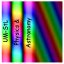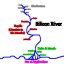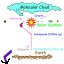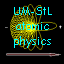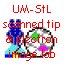For some related links:
• Browser-interactively solve your own constant-acceleration problems.
• Figure traveler time on a "1-gee" trip to Andromeda galaxy.
• Try focussing a high-res electron microscope image on-line!
• Does making a hotdog require 50 nanoseconds of life's power stream?
• How are Balinese candle-dancing and electron spins connected?
• Is statistical physics dead, or is there a paradigm change afoot?
• deBroglie's electrons and some amazing TEM facts.
• At UM-StLouis see also: a1toc, cme, fzx&astr, progs, stei-lab, & wuzzlers.
• For source, cite URL at http://newton.umsl.edu/~philf/a1dwuzzl.html
• Copyright (1970-96) by Phil Fraundorf
• Version release date: 22 Mar 1996.
• Since 22 Feb 1997, you are visitor number [broken counter].
• Mindquilts site page requests ~2000/day approaching a million per year.
• Requests for a "stat-counter linked subset of pages" since 4/7/2005: .